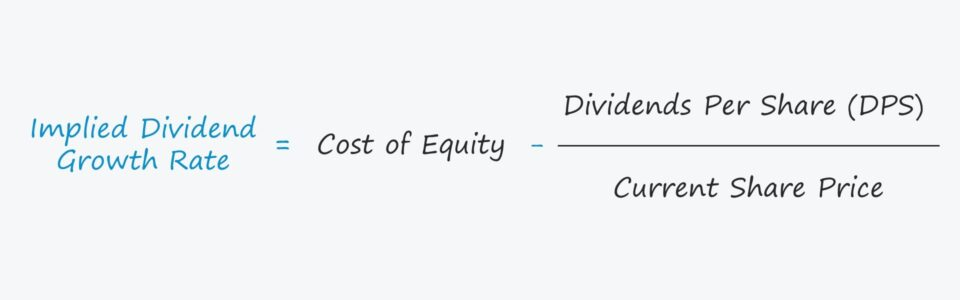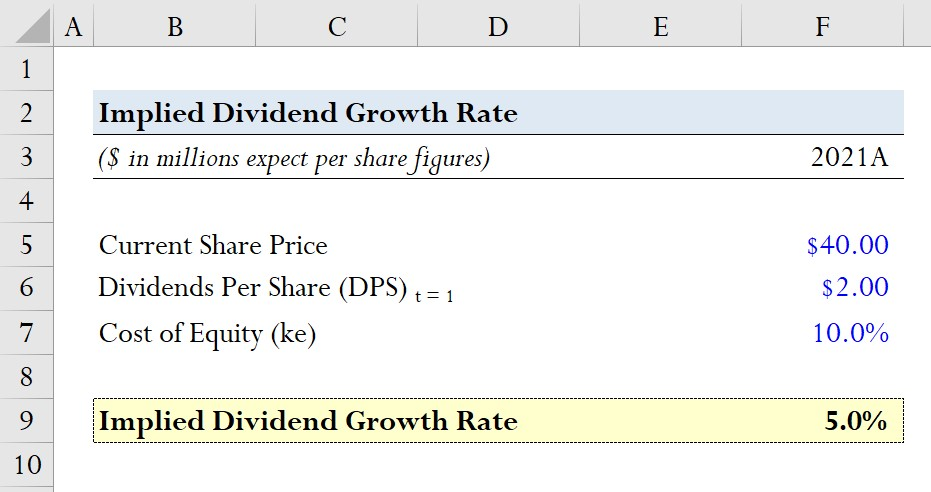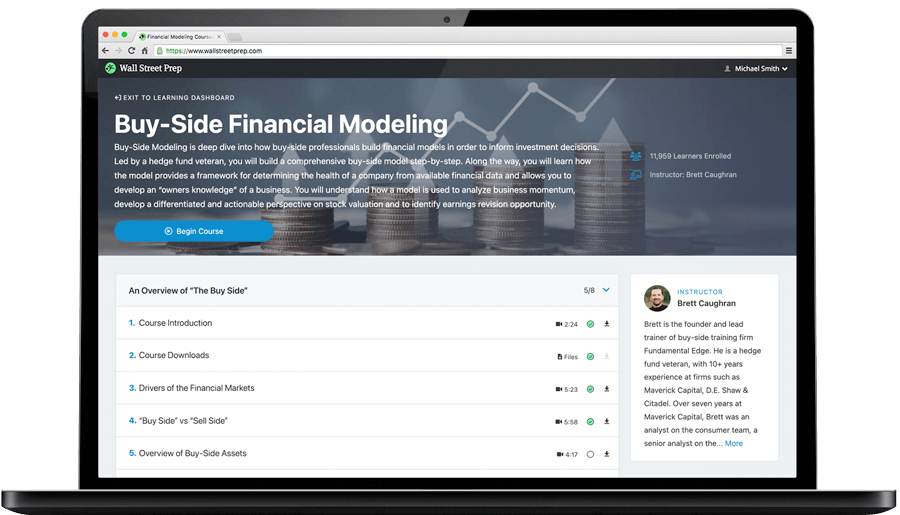Welcome to Wall Street Prep! Use code at checkout for 15% off.# Implied Dividend Growth Rate

Guide to Understanding the Implied Dividend Growth Rate## Implied Dividend Growth Rate Formula

The dividend discount model (DDM) states that the intrinsic value (and share price) of a company is determined by the sum of all of its future dividend issuances, discounted to the present date.

While the dividend discount model is typically used for estimating the fair value of a dividend-issuing company, the formula can be rearranged to back solve for the implied dividend growth rate, instead.

The simplest variation of the dividend discount model is the Gordon Growth Model, which assumes that dividends are anticipated to grow indefinitely at a constant rate.

The Gordon Growth Model approximates the share price of a company by taking the next period’s dividend per share (DPS) and dividing it by the required rate of return minus the dividend growth rate.

###### Gordon Growth Model (GGM) Formula
• Gordon Growth Model (GGM) = Next Period Dividends Per Share (DPS) ÷ (Cost of Equity – Dividend Growth Rate)

Since all variations of the dividend discount model treat dividend issuances as the cash flows of the company, the appropriate discount rate — i.e. the required rate of return — is the cost of equity (ke), which represents solely equity shareholders.

Normally, the formula above would be used to predict a company’s share price and to decide whether its shares are undervalued (or overvalued).

But we’ll do the reverse here in order to calculate the dividend growth rate, where we divide DPS by the current share price and subtract that amount from the cost of equity.

###### Implied Dividend Growth Rate Formula
• Implied Dividend Growth Rate = Cost of Equity – (Dividends Per Share ÷ Current Share Price)

## What is a Good Implied Dividend Growth Rate?

The dividend growth rate assumption is a key input in determining the fair value of a company’s shares in a dividend discount model.

But in order for the model to function properly, the growth rate must be less than the required rate of return, i.e. the discount rate assumption.

If the growth rate assumption exceeds the discount rate, the output from the model will be negative, which would result in a nonsensical conclusion.

The same reasoning applies to our modified model, where we’ll calculate the implied dividend growth rate, as opposed to the stock price.

With regard to interpreting the implied growth rate’s impact on the estimated intrinsic value of the company, the following rules are generally true:

• Higher Implied Growth Rate + Lower Discount Rate → Higher Valuation
• Lower Implied Growth Rate + Higher Discount Rate → Lower Valuation

## Implied Dividend Growth Rate Calculator

We’ll now move to a modeling exercise, which you can access by filling out the form below.#### Get the Excel Template!Submitting...

## Implied Dividend Growth Rate Calculation Example

Suppose a company is trading at a share price of \$40.00 as of the current date.

The expected dividend per share (DPS) next year is \$2.00 and the cost of equity, i.e. the required rate of return for shareholders, is 10.0%.

• Current Share Price = \$40.00
• Expected Dividend Per Share (DPS) = \$2.00
• Cost of Equity (ke) = 10.0%

Given those set of assumptions, we’ll calculate our implied growth rate by taking dividing our DPS (\$2.00) by the current share price (\$40.00) and then subtracting it from the cost of equity (10.0%).

• Implied Dividend Growth Rate = 10.0% (\$2.00 ÷ \$40.00) = 5.0%

We arrive at an implied growth rate of 5.0%, which we would then compare to the growth rate embedded in the current market share price to determine if the company’s shares are undervalued, overvalued, or priced near their fair value.Step-by-Step Online Course

### Learn Buy-Side (Hedge Fund) Modeling

Led by a former hedge fund PM (Maverick, Citadel, DE Shaw, Schonfeld), this program begins where financial modeling training ends — with a deep-dive into how buy-side analysts build financial models to make key investment decisions.